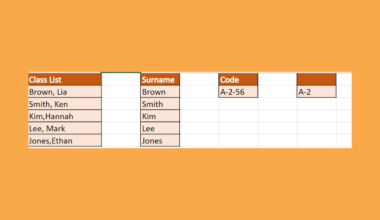# How to Calculate Percentage Increase in Excel

This guide will discuss how to calculate percentage increase in Excel

Calculating percentage increases on paper can be difficult, especially when dealing with large numbers. But calculating percentage increases and even decreases in Excel is simple.

A percentage refers to a number or value out of 100. For instance, 15% is simply 15 out of 100, and 56% is 56 out of 100. We don’t immediately get the percentage in Excel when inputting a number.

First, we can put the decimal value. Then, click the percentage symbol to apply a percent sign. For example, input 0.23 and apply the percentage format to make it 23%.

Second, we can get the percentage of a total in Excel. We simply divide the two values to get the decimal and apply the percentage format to the decimal.

For example, we want to obtain a percentage of 30 out of 50. We just need to divide the values so =30/50. And we would get the decimal 0.6. Then, apply the percent symbol to get our percentage of 60%.

Calculating percentage changes in Excel utilizes basic mathematics. It is simply a matter of subtraction, division, and the use of the SUM function for both percentage increase and percentage decrease.

Let’s take an example where you need to calculate the percentage increase in Excel.

Suppose you are making a sales report for January and February. And you want to emphasize the increase in sales in February. Let’s say you had a \$13500 sale in January and \$16780 in February. Using Excel to calculate the increase, you would get a 24% increase.

Awesome! Let’s dive into a real example of calculating a percentage increase in Excel.

## A Real Example of Calculating Percentage Increase in Excel

Calculating percentage changes doesn’t have to be done at work but can also be applied in our personal life. For instance, we want to know how much our house bills increased this month compared to the previous month.To get the percentage increase, we simply need to subtract the new value from our initial value. Then, we will use the SUM function for the subtracted value and divide it by the initial value.

So our formula would be `=SUM(initial value-new value)/initial` value. In this case, the formula would be `=SUM(C3-B3)/B3`.We can also apply the same formula in calculating the percentage decrease. Let’s say our house bills decreased this month compared to the previous month.

Simply use the same formula `=SUM(C10-B10)/B10` to get the percentage decrease.Finally, it’s time to learn how to calculate percentage increases in Excel.

## How to Calculate Percentage Increase in Excel

This section will explain the step-by-step process of calculating percentage increases in Excel. Additionally, we will also calculate the percentage decrease.

1. First, create a table for your dataset. Input your initial value and new value. Also, leave a column to input the percentage increase.2. Second, calculate the percentage increase using the formula `=SUM(initial value/new value)/initial` value. In this case, our formula would be `=SUM(C3-B3)/B3`. Then, press the Enter key to show the percentage increase.3. Third, copy or drag down the formula from the first row to the rest of the rows. This will perform the same formula on the other values and get the percentage increase.4. Then, apply the percent symbol. To do this, go to the Home tab. Click the Number Format dropdown menu and select Percentage.5. If the percentage has decimal places and you only want whole numbers, we can easily fix this. Simply right-click on the value. Then, select Number Format.6. In the Number Format window, change the Decimal places to zero. Finally, click OK to apply the changes.7. Let’s try to calculate the percentage decrease this time. It uses the same `=SUM(initial value/new value)/initial` value formula. In this case, input the formula `=SUM(C10-B10)/B10`. Then, press the Enter key to return the result.8. Next, apply the percentage format. Again, go to Home and click on the Number Format menu. Then, select Percentage. Alternately, we can right-click the value and select Number Format.9. In the Number Format window, select Percentage as the category. Also, make sure the Decimal places are set to zero. Finally, click OK to apply the format.10. And tada! You have successfully calculated the percentage increase and decrease in Excel.That’s pretty much it! Finally, you have learned how to calculate percentage increases in Excel. Furthermore, you learned how to calculate the percentage decrease as well. Now you can apply this learning in your own work and even in your personal life whenever you deal with percentage changes.

Are you interested in learning more about what Excel can do? You can now use the various other Microsoft Excel formulas available to create great worksheets that work for you. Make sure to subscribe to our newsletter to be the first to know about the latest guides and tutorials from us.Our goal this year is to create lots of rich, bite-sized tutorials for Google Sheets users like you. If you liked this one, you'll love what we are working on! Readers receive ✨ early access ✨ to new content.

##### You May Also Like## How to Use Exponential Smoothing in Excel

This guide will explain how to use exponential smoothing in Excel using the data analysis tool. Excel is…## How to Multiply Column by a Constant in Excel

This guide will discuss how to multiply a column by a constant in Excel using three easy and…## How to Sum If Less Than in Excel

This guide will discuss how to sum if less than in Excel using the SUMIF function. The rules…## How to Create a Population Pyramid Chart in Excel

A population pyramid chart is useful when you indeed to illustrate the distribution of a population by age…